Chrome extensions by categories

174 results. Page 1 of 7.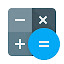#1. Calculator (v2.2.8)
A calculator with 29-functions for basic arithmetic, algebra, trigonometry and discrete math.…
1,724 10,000,000+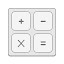#2. Calculator (v4.5.5)
Calculator for Chrome!
717 1,000,000+#3. Calculator (v1.1.0)
Scientific Calculator / Calc
1,659 400,000+Powerful calculator and converter for Chrome. The easiest way to calculate. Works offline
2,937 300,000+A powerful, programmable, scientific calculator.
699 300,000+#6. CircuitLab (v0.0.0.8)
Schematic editor and circuit simulator.
473 100,000+#7. MathStudio (v6.4)
Math for the modern web browser.
31 100,000+#8. Currency Converter (v1.0.4)
Convert your money in an easy way based on hourly updated currency exchange rates.
172 30,000+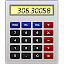#9. Melanto Calculator (v4.0.0.0)
One different calculator app...
138 20,000+#10. Unit Convertor (v1.9)
A must have utility that helps you convert between different units of measurement.
76 20,000+#11. Calculator (v3.1.0.0)
One different scientific calculator (desktop version)...
40 20,000+Free calculator, unit converter for general, students, Mathematics, Engineering. Easy-to-use General or Scientific Calculator.
43 20,000+#13. Simple Calc (v2.0.1)
Simple calculator with advanced features. Offline and panel mode support.
40 10,000+#14. Simple Calculator (v1.3.2)
Just a Calculator, Simple to use, and 100% offline
23 10,000+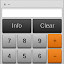#15. Basic Calculator (v0.1.1)
A calculator to perform simple math operations. Source code: https://github.com/baclap/calculator
12 10,000+#16. EdisonCloud (v1.0.0.6)
Web-based 3D Lab for Exploring Electricity & Electronics
15 9,000+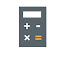#17. Calculator XL (v0.8)
Calculator XL gives you the gold standard of calculator with big buttons!
33 8,000+If you work in the wireless networking industry then this app will help you out.
70 7,000+#19. AV Tools (v1.4.2)
Be an audio visual pro wherever you go with AV Tools
40 7,000+#20. TINACloud (v1.0.0.24)
Web-based Circuit Design and Analysis
31 4,000+A programmer's calculator that supports 8, 16, 32, and 64-bit signed and unsigned numbers.
15 4,000+#22. Calculator (v2.0.0)
A simple calculator that remembers your last calculation. You can close it at any time and reopen it without losing your work.
6 4,000+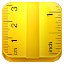#23. Unit Converter (v1.0.4)
Convert units in easy way.
11 3,000+#24. RPN Calculator (v1.0.0.4)
A simple RPN calculator application; opens in a popup window.
31 2,000+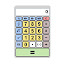#25. Calculator (v1.1)
Powerful calculator and converter for Chrome. The easiest way to calculate.
21 2,000+
174 results. Page 1 of 7.